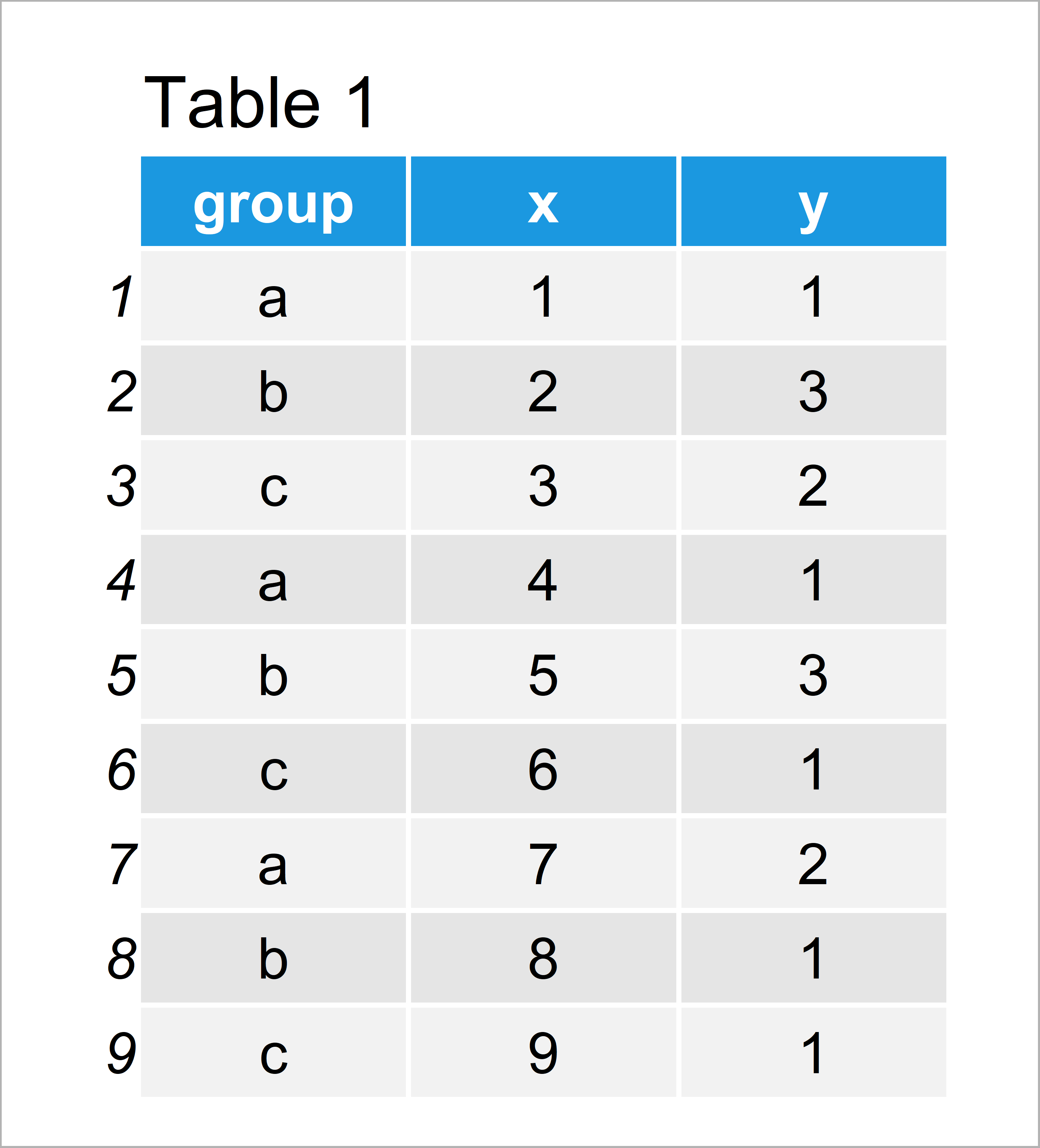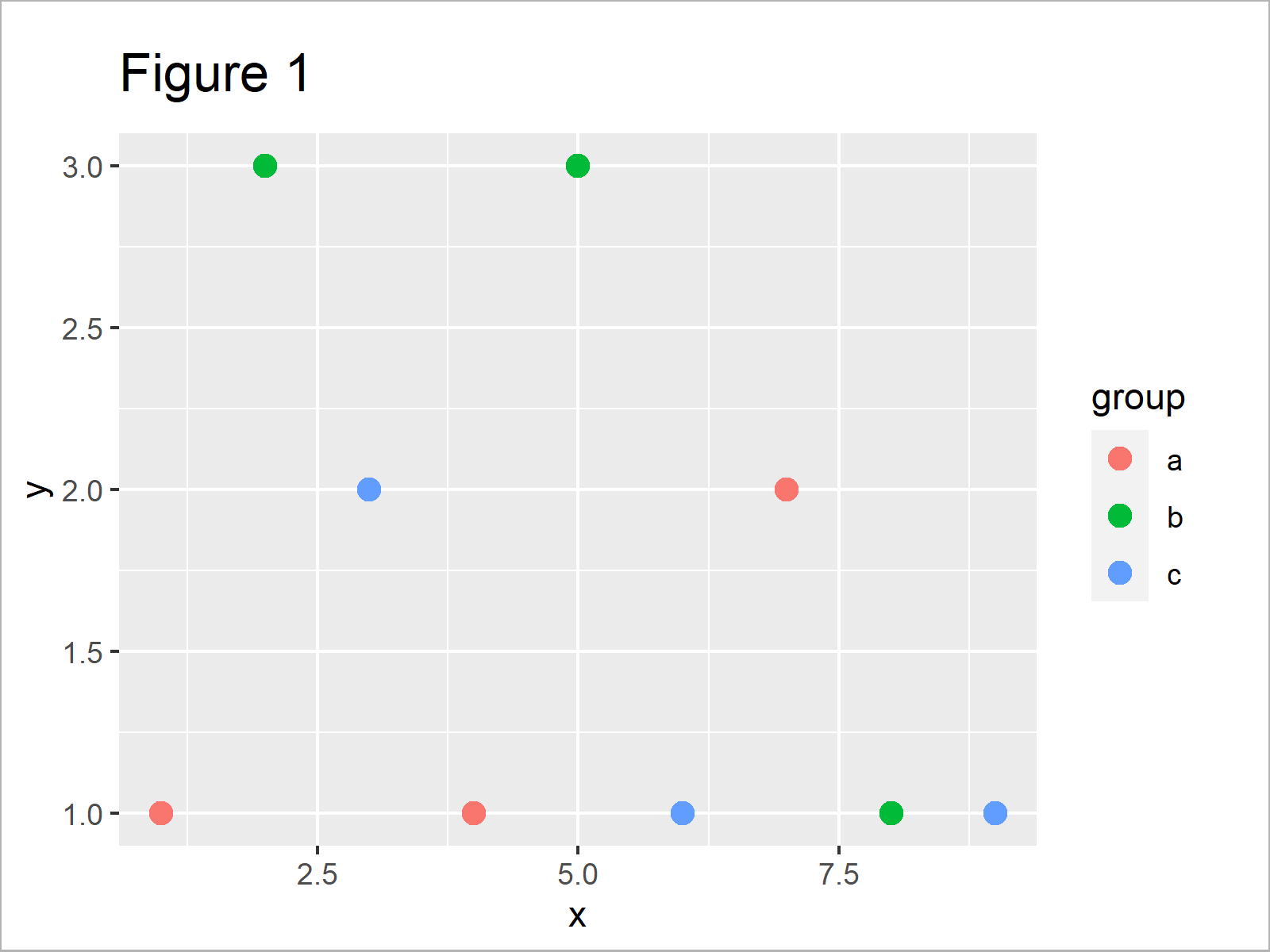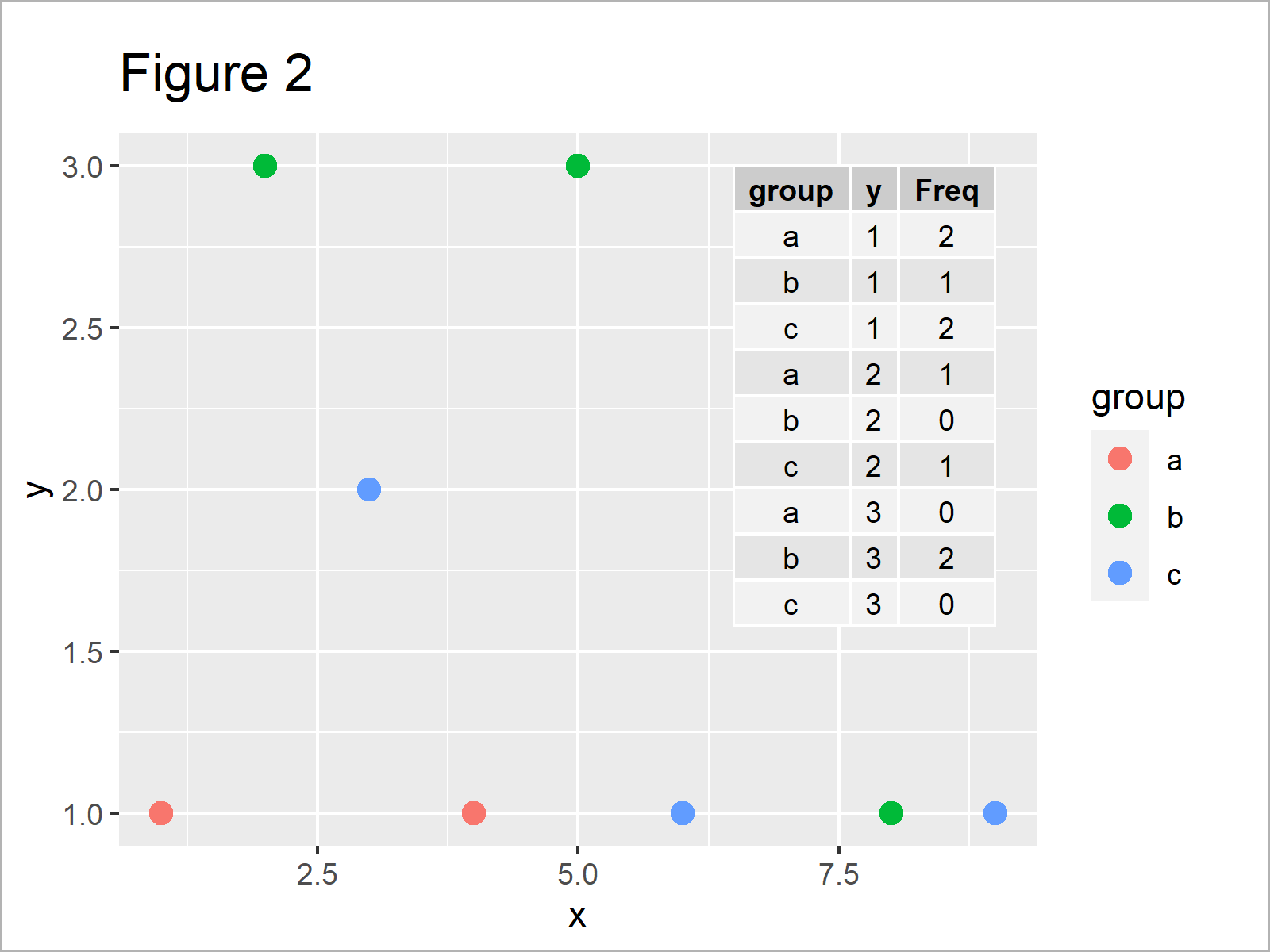# Add Table to ggplot2 Plot in R (Example)

In this R article you’ll learn how to draw a data frame table to a ggplot2 plot.

The post is structured as follows:

Let’s just jump right in.

## Example Data, Add-On Packages & Default Plot

The following data will be used as basement for this R tutorial:

```my_data <- data.frame(group = letters[1:3],         # Create example data
x = 1:9,
y = c(1, 3, 2, 1, 3, 1, 2, 1, 1))
my_data                                             # Print example data```Table 1 visualizes the output of the RStudio console and reveals that our example data has nine data points and three columns.

To be able to use the functions of the ggplot2 package, we also need to install and load ggplot2:

```install.packages("ggplot2")                         # Install ggplot2 package

As next step, we can create a plot of our data:

```ggp <- ggplot(my_data, aes(x, y, color = group)) +  # Create ggplot2 plot without table
geom_point(size = 3)
ggp                                                 # Draw plot```By running the previous R syntax we have created Figure 1, i.e. a ggplot2 scatterplot without any table in the plotting area.

Let’s create a data frame table in R:

```my_table <- as.data.frame(table(my_data[ , c(1, 3)])) # Create example table
my_table                                            # Print example table```As shown in Table 2, the previous code has created a data frame with three columns.

In the following example, I’ll explain how to add this table to our graph using the ggplot2 and ggpmisc packages.

## Example: Add Table to ggplot2 Plot Using ggpmisc Package

This example explains how to add a table to a ggplot2 plot in R.

First, we have to install and load the ggpmisc package:

```install.packages("ggpmisc")                         # Install & load ggpmisc
library("ggpmisc")```

Next, we can use the annotate function to add our table within the plot window of our graph:

```ggp +                                               # Add table to ggplot2 plot
annotate(geom = "table",
x = 9,
y = 3,
label = list(my_table))```By executing the previous code we have drawn Figure 2, i.e. a ggplot2 scatterplot with a table in the plotting area.

## Video & Further Resources

Would you like to know more about ggplot2 plots and tables? Then you could watch the following video of my YouTube channel. I’m illustrating the topics of this article in the video:

Please accept YouTube cookies to play this video. By accepting you will be accessing content from YouTube, a service provided by an external third party.If you accept this notice, your choice will be saved and the page will refresh.

Besides the video, you could have a look at the other articles on Statistics Globe. I have released numerous other articles already.

This tutorial has illustrated how to add a table within the plotting area of a ggplot2 graphic in the R programming language. Don’t hesitate to let me know in the comments, in case you have additional questions.

Subscribe to the Statistics Globe Newsletter

•Fernando Ramires
March 3, 2021 1:02 pm

In the line where you create the table it is necessary to correct the data name from “data” to “my_data” .. so the code will work.

•March 3, 2021 1:22 pm

Thanks a lot for this hint Fernando! I just corrected the code.

Thanks

Joachim

•JT
October 7, 2022 5:20 pm

how do you adjust the location of where the table is inserted on the graph?

•November 14, 2022 11:55 am

Hey JT,

Please excuse the late response. I was on a long holiday so unfortunately I wasn’t able to reply sooner. Still need help with your code?

Regards,
Joachim

•Carly Andrews
December 1, 2022 7:14 pm

Hi! Is it possible to change the font size of the table?

•December 14, 2022 9:45 am

Hello Carly,

Sorry for the late response. If you haven’t solved the problem yet, you can try the following code below. I have added the “size” argument in the annotate function.

```ggp +                                               # Add table to ggplot2 plot
annotate(geom = "table",
x = 9,
y = 3,
label = list(my_table), size=8)```

Regards,
Cansu

•Emmanuel Jjunju
July 12, 2023 1:19 pm

can you assign different colours for each table column?

•July 13, 2023 8:00 am